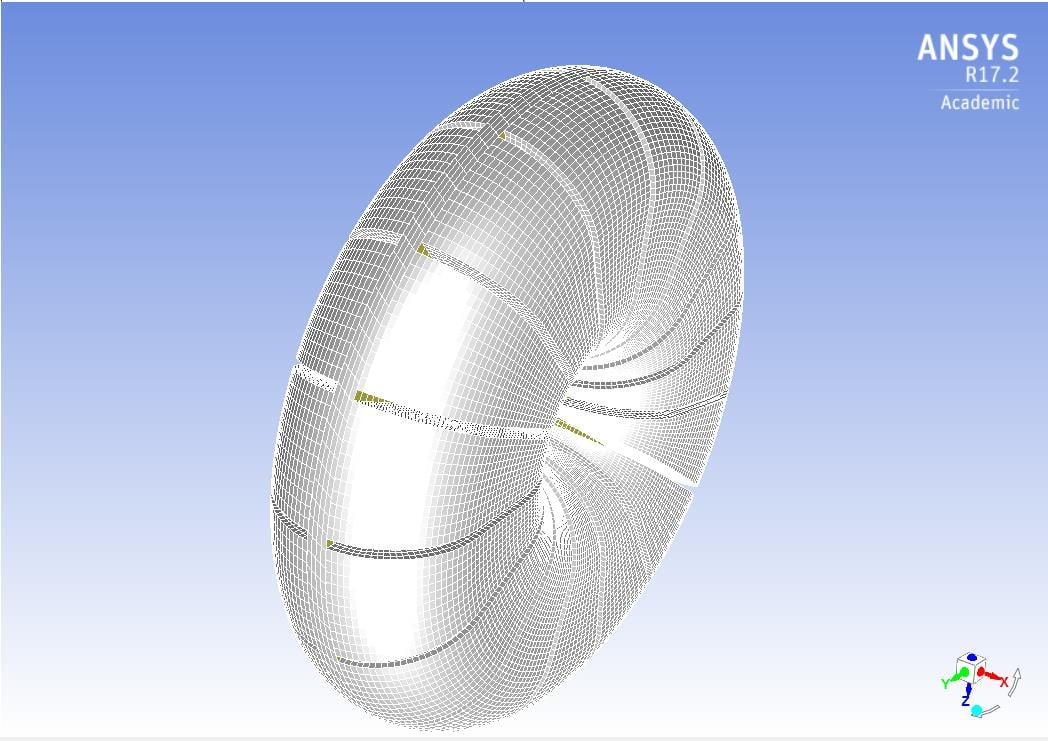## Fluids

Topics relate to Fluent, CFX, Turbogrid and more

•sdarmayuda
Subscriber

Hallo,

I'm quite new and still learning this program ANSYS 17.2 academic version. I'm trying to simulate a hydrodynamic coupling. It consists of two part, Pump side and Turbine side (A screenshoot of the model is available). The rotational velocity of the pump side is constant. The rotational velocity of the turbine side is changing in respect of time and depends on the value of the working torque (from the fluid to the turbine's blade).

I've made a plane on my turbine's geometry and the working torque (Moment) on the turbine can displyed using 'monitors' and 'reports'.

And now I'm going to use this value, so the turbine's rotational velocity can change in respect of time.

1. How can I Import this value into my UDF? Which variable are used in this case?

2. And will this UDF File be read in every Iteration? Or will it be just be read once? I dont know if I should put the looping here.

3. While I was trying to Interpret my UDF File, this warning came 'line 23: structure reference not implemented'. Can someone Show/tell me the mistakes?

Here is my UDF File.

***********************************************************

#include "udf.h"
#define Niter 25 /* iteration update interval */
#define omg_ts 0.0 /* starting omega */
#define omg_tf 600.0 /* final omega */
#define Jt 0.000554 /* Moment Inertia of the Turbin*/

{
int zoneID;
int iter = (nres==0)?(1)(int)count2[nres-1]);
float omgt;

zoneID = 2;

if ( (iter%Niter)==0 )
{
del_omgt = Mt/Jt;
omgt = omg_ts + del_omgt*(iter/Niter);
if ( omgt > omg_tf ) omgt = omg_tf;
}
}
************************************************************

I'm new and therefore I really appreciate any Reply and any help. Thanks in advance.

Best regards,

Darma Yuda•sdarmayuda
Subscriber

I have once again adjusted my UDF. But still I can't find a way to retrieve the value of the working torque. Can someone give me a hint about this? Thank you very much

************************************************************************************

#include "udf.h"

DEFINE_TRANSIENT_PROFILE(testudf,CURRENT_TIME)

{

real omega_ts=0.0; /*starting omega*/

real omega_tf=600.0; /*final omega*/

real Jt=0.000554; /*Moment Inertia of the Turbin*/

real delta_t=0.0001; /*delta t simulation*/

real CURRENT_TIMESTEP;

real CURRENT_TIME;

real alpha_t; /*angular acceleration*/

cell_t c;

begin_c_loop (c,t)

{

alpha_t= Mt/Jt; /*M = J*alpha, but how can I retrieve the value of Mt?*/

omega_ts+=alpha_t*CURRENT_TIMESTEP;

if (omega_ts > omega_tf)

{omega_ts = omega_tf;}

}

end_c_loop (c,t)

}

•sdarmayuda
Subscriber

Hallo everyone,

I have found how to retrieve the torque from Fluent. However, there is still error in the code after I intepreted the UDF code. it says "line 14: parse error". I think it is about the macro DEFINE_TRANSIENT_PROFILE. Can somebody help to show me the mistakes about this macro? Thank you very much.

Here is my UDF Code:

**************************************************************************

#include "udf.h"

DEFINE_ON_DEMAND(wall_forces)

Domain * domain = Get_Domain (1); /* For multiphase flow, you need to set the Sub domain */

real CG, force, moment;

t = Lookup_Thread (domain, 13); /* 13 is the ID of the wall to be determined. */

NV_S (CG, =, 0.0); /* coordinates of the center position to find the moment. */

Compute_Force_And_Moment (domain, t, CG, force, moment, TRUE);

Message("f=(%e, %e, %e), m=(%e, %e, %e)n", force, force, force, moment, moment, moment);

/* force , force , force  are respectively x, y, z-direction forces, Is the sum of the force by the force and the shear stress due to pressure. */

}

DEFINE_TRANSIENT_PROFILE(testudf, CURRENT_TIME)

{

real omega_ts=0.0; /*starting omega*/

real omega_tf=600.0; /*final omega*/

real Jt=0.000554; /*Moment Inertia of the Turbin*/

real delta_t=0.0001; /*delta t simulation*/

real CURRENT_TIMESTEP;

real CURRENT_TIME;

real alpha_t; /*angular acceleration*/

cell_t c;

t=Lookup_Thread(domain,9); /*Cell Zone ID = 9*/

begin_c_loop (c,t)

{

alpha_t= 15*moment/Jt; /*there are 15 blades, x axis as rotation axis*/

omega_ts+=alpha_t*CURRENT_TIMESTEP;

if (omega_ts > omega_tf)

{omega_ts = omega_tf;}

}

end_c_loop (c,t)

}